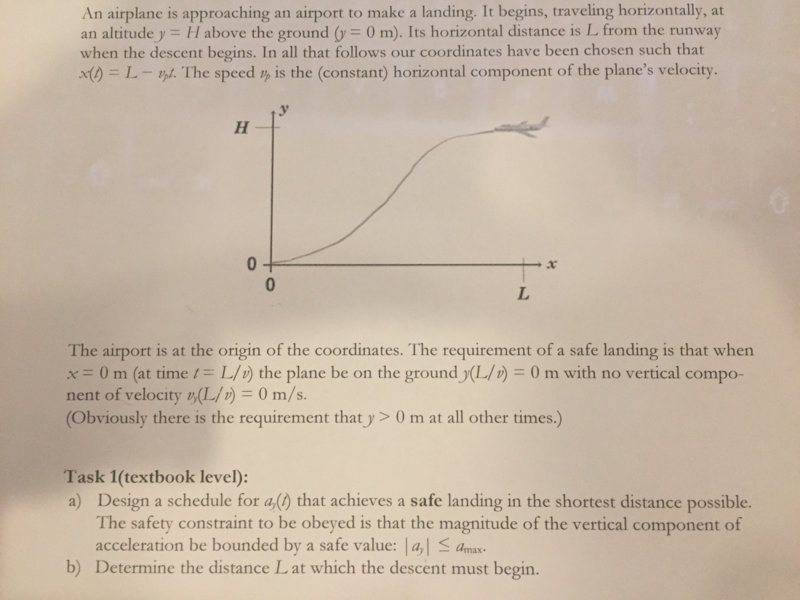# Relevant equations for an airplane landing

The problem

I'm pasting the problem below mainly for the plot of y vs x.A possible way to think of it

I suppose the important thing here is to find y(t) with the condition that -amax<ay<amax, and vy(L/vp)=0
My attempt

I've modelled acceleration as a(t)=-kt and got an equation for y(t) as a function of t3 which can be replaced by L/v. But the problem is that, plotting on gnuplot, the solution doesn't convince me. Would you give me some hints on how to model a(t), please?

#### Attachments

Last edited:

Orodruin
Staff Emeritus
Homework Helper
Gold Member
2021 Award
You have a maximal bound on the allowed acceleration. How can you use this to go down as fast as possible if you do not care about having ##v_y = 0## when you land?

You have a maximal bound on the allowed acceleration. How can you use this to go down as fast as possible if you do not care about having ##v_y = 0## when you land?
Writing v(t)=-t2/2 I don't see any conclusion (v(0)=0). All I can see is that |v(t)| will increase continuously and of course that's wrong because at t=L/v, vy=0

Last edited:
Orodruin
Staff Emeritus
Homework Helper
Gold Member
2021 Award
No, you are just making an assumption that ##a = -kt##. There are no grounds for making this assumption and it leads to a false result. For times ##t < a_0/k##, it will not accelerate enough - leading to a slower descent than possible - and for ##t > a_0/k## it violates the requirement that ##|a| < a_0##.

No, you are just making an assumption that ##a = -kt##. There are no grounds for making this assumption and it leads to a false result. For times ##t < a_0/k##, it will not accelerate enough - leading to a slower descent than possible - and for ##t > a_0/k## it violates the requirement that ##|a| < a_0##.
Fine but acceleration is function of time, I just tried the simplest. Cause, for example, a(t)=1/t seems more complex. Do you see any way to solve it?

Orodruin
Staff Emeritus
Homework Helper
Gold Member
2021 Award
Fine but acceleration is function of time, I just tried the simplest.
No you did not try the simplest. There is nothing stating that the acceleration must be continuous or given by an easy functional expression.

No you did not try the simplest. There is nothing stating that the acceleration must be continuous or given by an easy functional expression.
But if we state that a=amax wouldn't be wrong? I mean, it must change with time (at least its sign).

No you did not try the simplest. There is nothing stating that the acceleration must be continuous or given by an easy functional expression.
Oh I see. Do you mean it should be splitted up? But in that case it'd be quite unreal/unphysical.

Orodruin
Staff Emeritus
Homework Helper
Gold Member
2021 Award
But in that case it'd be quite unreal/unphysical.
While it is true that you probably cannot change the acceleration instantly from one value to another, you can get close enough to it for it not to matter as long as the overall time-scale of the descent is much longer than the time it takes to reverse the acceleration.

After some more trials this is what I've got. For t>tc which is the time where acceleration change its sign,

v(t)=-amax*tlim+ amax*(t-tl)/
and
y(t)= -amax*t2lim+ amax*(t-tl)2/

as t=L/vp, replacing into t, deriving respect to L, and solving for L, it yields:

L=tc*vp

Any suggestion?

v(t)=-amax*tlim+ amax*(t-tl)/
and
y(t)= -amax*t2lim+ amax*(t-tl)2/

Here you are guessing that the quickest way down is max acceleration down until at some point you apply max acceleration up. I think that is right. However then you somehow put this together with the horizontal motion and L. Your equations are in the y dimension. Stay there a minute.

Look at the first equation. They told you vy had to be zero when you reach the ground. What does that tell you about the two intervals?

Now look at your second equation. The initial vertical velocity is zero, so you don’t need a v0 t term for the first segment of descent. But is that true for the second segment? As you have it written the plane will wind up right back where it started.

•santimirandarp
Good points.

1) I think is correct because t=L/v, time of flight is independent of the dimension.

2) The time intervals of acceleration are identical, that follows from v(t).

3) The integration was wrong y(t)= yl -amax*tl*(t-tl)+ amax*(t-tl)2/2

I'm not sure of this last step. So tired of this problem.

3) The integration was wrong y(t)= yl -amax*tl*(t-tl)+ amax*(t-tl)2/2

Still not right I’m afraid. Try thinking of it as two problems. First from H to H/2 with a down, then take the result as the initial conditions (position and velocity) for H/2 to 0 with a up.

Adding yl=H/2 it'd be complete isn't it?

Adding yl=H/2 it'd be complete isn't it?

Actually, I think I was wrong. That wasn’t what was bothering me. I didn’t think the second term looked right. However, looking at it again I can’t figure out what I even thought was wrong. It looks ok to me. Sorry about that.

Adding yl=H/2 it'd be complete isn't it?

In fact, hallelujah, it leads directly to where I would have started the problem. You have an expression for y(t) in the second half of the motion. You will definitely want that H/2 in there. Now you know when the plane lands y(t) = 0. Is there anything you can say about t at that time compared to tl?

haruspex